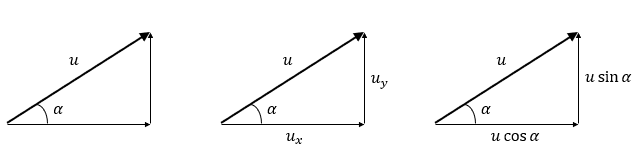# Projectiles

A LevelAQAEdexcelOCR

## Projectiles

We model projectile motion in two components, horizontal and vertical.

Make sure you are happy with the following topics before continuing.

A LevelAQAEdexcelOCR

## Splitting Velocity into Components

Using trigonometry, we convert a standard projectile motion into its two components.

Generally, we have a particle fired with a velocity $u$ at an angle of $\textcolor{orange}{\alpha}$, which gives

Horizontal:

$u\cos \textcolor{orange}{\alpha}$

Vertical:

$u\sin \textcolor{orange}{\alpha}$From here, we can use either method of modelling motion – SUVAT or integration/differentiation. We should use these piecewise, meaning, our equations in the vertical component are not the same equations in the horizontal component.

A LevelAQAEdexcelOCR

## Finding a Maximum Height and Maximum Velocity

Remember, we can also find a maximum or minimum displacement by differentiating and finding the time $\textcolor{purple}{t}$ where the velocity of our object is $0$.

We can also find a maximum or minimum velocity by differentiating again and finding a time $\textcolor{purple}{t}$ where the acceleration, $\textcolor{blue}{a} = 0$.

A LevelAQAEdexcelOCR
A LevelAQAEdexcelOCR

## Example: Projectiles in Vector Notation

We can also use vectors to make projectile motion much neater.

So, for example, say a ball is thrown off of a cliff with a velocity of $(15\textbf{i} + 7\textbf{j})\text{ ms}^{-1}$ with $\textbf{i}$ its horizontal velocity, and $\textbf{j}$ its upward vertical velocity. Assume that the ball accelerates due to gravity and experiences no air resistance. Given it is in the air for $\textcolor{purple}{t} = \textcolor{purple}{5}\text{ seconds}$, how tall is the cliff, what horizontal distance does the ball travel and what is its final velocity?

Assume $g = 10\text{ ms}^{-2}$.

[4 marks]

$\textcolor{limegreen}{\underline{s}} = \underline{u}\textcolor{purple}{t} + \dfrac{1}{2}\textcolor{blue}{\underline{a}}\textcolor{purple}{t}^2$

gives

$\textcolor{limegreen}{\underline{s}} = \textcolor{purple}{5}(15\textbf{i} + 7\textbf{j}) + \dfrac{\textcolor{purple}{25}}{2}(\textcolor{blue}{-10\textbf{j}}) = \textcolor{limegreen}{75\textbf{i} - 90\textbf{j}}$

So, the ball travels $\textcolor{limegreen}{75}\text{ m}$ horizontally, and the cliff is $\textcolor{limegreen}{90}\text{ m}$ tall.

$\textcolor{red}{\underline{v}} = \underline{u} + \textcolor{blue}{\underline{a}}\textcolor{purple}{t}$

gives

$\textcolor{red}{\underline{v}} = (15\textbf{i} + 7\textbf{j}) - (\textcolor{blue}{10} \times \textcolor{purple}{5})\textbf{j} = \textcolor{red}{15\textbf{i} - 43\textbf{j}}\text{ ms}^{-1}$

A LevelAQAEdexcelOCR

## Projectiles Example Questions

$\underline{u} = 5$ gives

Horizontally:

$5\cos 60° = 2.5\text{ ms}^{-1}$

Vertically:

$5\sin 60° = 4.33\text{ ms}^{-1}\text{ (to }2\text{ dp)}$

Using

$v = u + at$

we have

$0 = 14.7 - 9.8t$

giving

$t = 1.5\text{ seconds}$

Substituting this into

$s = ut + \dfrac{1}{2}at^2$

we can prove that

\begin{aligned}s&=(14.7 \times 1.5) + \left( \dfrac{1}{2} \times -9.8 \times 1.5^2\right)\\[1.2em]&=11.025\text{ m}\end{aligned}

which is greater than $11\text{ m}$, as required.

$\underline{u} = (30\textbf{i} + 24.5\textbf{j})$

and

$\underline{a} = (-2\textbf{i} - 9.8\textbf{j})\text{ ms}^{-2}$

Using $\underline{s} = \underline{u}t + \dfrac{1}{2}\underline{a}t^2$ gives

$125\textbf{i} = (30t\textbf{i} + 24.5t\textbf{j}) + (-t^2\textbf{i} - 4.9t^2\textbf{j})$

Meaning

$125 - 30t + t^2 = 0$

and

$24.5t - 4.9t^2 = 0$

This gives $t = 5\text{ seconds}$.

A Level

A Level

## You May Also Like...### MME Learning Portal

Online exams, practice questions and revision videos for every GCSE level 9-1 topic! No fees, no trial period, just totally free access to the UK’s best GCSE maths revision platform.

£0.00

A Level